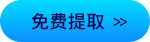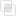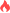2021-04-07 14:32:53641浏览 · 0收藏 · 0评论1、说明

array_slice()返回数组中指定下标offset和长度标记的子数组切片。

2、语法

`array_slice(array,start,length,preserve)`

3、参数

array

start

length

Preserve

4、实例

```<?php
\$input = array("a", "b", "c", "d", "e");

\$output = array_slice(\$input, 2);   // returns "c", "d", and "e"
\$output = array_slice(\$input, -2, 1); // returns "d"
\$output = array_slice(\$input, 0, 3);  // returns "a", "b", and "c"

print_r(array_slice(\$input, 2, -1)); // array(0 => 'c', 1 => 'd');
print_r(array_slice(\$input, 2, -1, true)); // array(2 => 'c', 1 => 'd');```php数组的分类有哪几个php数组排序的函数有几个php数组中的二分查找是什么php数组排序函数php数组中二分查找是什么php数组如何进行堆栈的模拟php数组如何转换为xml的形式？php数组交集函数php数组赋值方式php数组添加元素python Pool常用函数有哪些827

python中进程池Pool的初始化387

python中Queue如何通信751